Download Presentation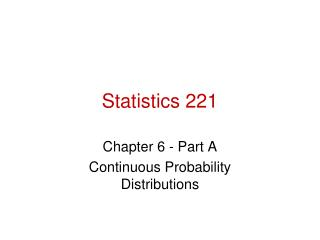Statistics 221

# Statistics 221 - PowerPoint PPT Presentation

Statistics 221 Chapter 6 - Part A Continuous Probability Distributions Continuous variables and their probabilitiesI am the owner, or an agent authorized to act on behalf of the owner, of the copyrighted work described.
Download Presentation## Statistics 221

An Image/Link below is provided (as is) to download presentation

Download Policy: Content on the Website is provided to you AS IS for your information and personal use and may not be sold / licensed / shared on other websites without getting consent from its author.While downloading, if for some reason you are not able to download a presentation, the publisher may have deleted the file from their server.

- - - - - - - - - - - - - - - - - - - - - - - - - - E N D - - - - - - - - - - - - - - - - - - - - - - - - - -
Presentation Transcript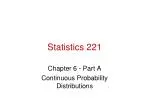### Statistics 221

Chapter 6 - Part A

Continuous Probability Distributions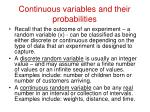Continuous variables and their probabilities
• Recall that the outcome of an experiment – a random variable (x) - can be classified as being either discrete or continuous depending on the type of data that an experiment is designed to capture.
• A discrete random variable is usually an integer value – and may assume either a finite number of values or an infinite sequence of values. Examples include: number of children born or number of customers arriving.
• A continuous random variable can be any real number in an interval or collection of intervals. Examples include: weights, distance, and time.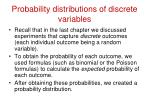Probability distributions of discrete variables
• Recall that in the last chapter we discussed experiments that capture discrete outcomes (each individual outcome being a random variable).
• To obtain the probability of each outcome, we used formulas (such as binomial or the Poisson formulas) to calculate the expected probability of each outcome.
• After obtaining these probabilities, we created a probability distribution.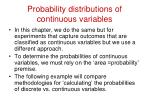Probability distributions of continuous variables
• In this chapter, we do the same but for experiments that capture outcomes that are classified as continuous variables but we use a different approach.
• To determine the probabilities of continuous variables, we must rely on the ‘area =probability’ premise.
• The following example will compare methodologies for ‘calculating’ the probabilities of discrete vs. continuous variables.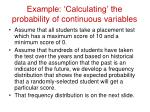• Assume that all students take a placement test which has a maximum score of 10 and a minimum score of 0.
• Assume that hundreds of students have taken the test over the years and based on historical data and the assumption that the past is an indicator of the future, we develop a frequency distribution that shows the expected probability that a randomly-selected student will get a particular score.
• That frequency distribution is on the next slide.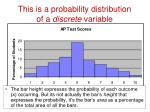This is a probability distributionof a discrete variable
• The bar height expresses the probability of each outcome (x) occurring. But its not actually the bar’s height that expresses the probability, it’s the bar’s area as a percentage of the total area of all the bars.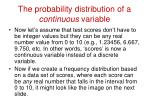The probability distribution of a continuous variable
• Now let’s assume that test scores don’t have to be integer values but they can be any real number value from 0 to 10 (e.g., 1.23456, 6.667, 9.750, etc. In other words, ‘scores’ is now a continuous variable instead of a discrete variable.
• Now if we create a frequency distribution based on a data set of scores, where each score can be any real number that falls in the interval from 0 to 10, it might look like the image on the next slide.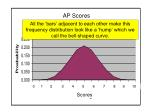AP Scores

All the ‘bars’ adjacent to each other make this frequency distribution look like a ‘hump’ which we call the bell-shaped curve.

0 1 2 3 4 5 6 7 8 9 10

Scores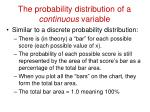The probability distribution of a continuous variable
• Similar to a discrete probability distribution:
• There is (in theory) a “bar” for each possible score (each possible value of x).
• The probability of each possible score is still represented by the area of that score’s bar as a percentage of the total bar area.
• When you plot all the “bars” on the chart, they form the total bar area.
• The total bar area = 1.0 meaning 100%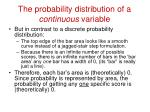The probability distribution of a continuous variable
• But in contrast to a discrete probability distribution:
• The top edge of the bar area looks like a smooth curve instead of a jagged-stair step formulation.
• Because there is an infinite number of possible scores, there is an infinite number of bars in the ‘bar area’ any one bar has a width of 0, (its “bar” is really just a line).
• Therefore, each bar’s area is (theoretically) 0. Since probability is represented by area, the probability of getting any one specific score is (theoretically) 0.‘Calculating’ probabilities of continuous variables
• Finding the probability of getting any particular x (e. g. test score) when x is a continuous variable is accomplished by finding the area of an interval under the curve line.
• Let’ say you want to find the probability of getting a score of 5 on the test.
• But we just learned that the probability of any one specific score is 0. Therefore:

P(x = 5.0) = 0

• Therefore, we must approximate the P(x=5) by finding the P(4.9 < x < 5.1).AP Scores

We find the probability that (4.9 < x < 5.1) by finding the percentage that the area in yellow is of the total bar area.

0 1 2 3 4 5 6 7 8 9 10

Scores

The total bar area = 100% or 1.0. What percentage is the yellow area of the total bar area? That’s the probability that 4.9 < x < 5.1.How do we find this area?
• If our probability distribution had a ‘flat top’, it would be easy, because the area would be a rectangle, and we could find the area by multiplying the width times the height.
• A probability distribution with a flat top all the way across is called a uniform distribution: every outcome value has the same chance of occurrence (like rolling a single die).
• Before we answer the question of what is P(4.9 < x < 5.1), let’s ask and answer a more simple question.Let’s say class length is a continuous variable with a uniform distribution

Every ending time between 50 and 52 minutes is equally probable.Figure 5-3

• To calculate probabilities of continuous variables, we calculate the area of the ‘bars’ as a percentage of the total area under the curve line.
• Since the area from 51.5 to 52 is ¼ of the total area, the probability that x >= 51.5 is 25%.

.5

P(x >=51.5) = .25The normal distribution (does not have a flat top)
• But for most variables, the probability distribution does not have a ‘flat top’ but instead looks like a bell curve:

A distribution that has a symmetric, bell-shape is called a ‘normal’ distribution.Characteristics of the normal distribution
• Many variables are known to have this shape of distribution (heights, weights, test scores, rainfall, etc.)
• The mean is at the highest point of the curve. The mean, median, and mode are equal.Characteristics of the normal distribution
• The distribution is symmetric; 50% of the possible outcome values lie to the left of the mean and 50% of the possible outcome values lie to the right of the mean.
• The tails extend to infinity in both directions but never actually touch the horizontal axis.Characteristics of the normal distribution
• The standard deviation determines how wide the curve is. A distribution curve with a low standard deviation will be more pointed and narrow that a distribution curve with a high standard deviation indicating more variation in the underlying data set.Characteristics of the normal distribution
• The total area (of the bars) under the curve line is 100%.
• Recall the empirical rule that states that:
• 68% of the area/possible outcome values will be within 1 std. deviation of the mean,
• 95% of the area area/possible outcome values will be within 2 std deviations of the mean and
• 99.7% of the area / possible outcome values will be within 3 std deviations of the mean.The formula for finding an area under a curve when the curve line is not flat
• If the frequency distribution was uniform, you can just multiply the height times the width to get an area of a ‘bar’.
• But when the frequency distribution is normal (as most are), you must use this probability density formula to find the area of an interval under the curve:
• Where:
• = the mean  = std. deviation  = 3.14159 e = 2.71828If we were to use the probability density function
• We would solve for f(x) when x= 4.9, then we would solve for f(x) when x=5.1.
• Then we would subtract the f(4.9) from f(5.1) to get the area under the curve line in between 4.9 and 5.1.
• That area would be expressed as a percentage of the total area under the curve.
• Since area = probability, if that area was, say 12%, then there would be a 12% chance that a randomly-selected student would get a score that was >4.9 and also < 5.1.Example 1
• The sitting height (from seat to top of head) of drivers must be considered in the design of a new car model. Men have sitting heights that are normally distributed with a mean of 36.0 and standard deviation of 1.4 inches. Engineers have provided plans that can accommodate men with sitting heights up to 38.8 inches but taller men cannot fit. If a man is randomly selected, find the probability that he has a sitting height less than 38.8 inches. Based on that result, is the current engineering design feasible?What is the P(x < 38.8)?
• This question can be simplified down to “what is the probability that a randomly-selected male individual will have a sitting height (x) that is less than 38.8 inches…
• …given that  = 36 and  = 1.4 inches?”
• Recall that probability can be found by finding the area of an interval under a probability distribution curve.1. Draw a picture to visualize the area/probability you’re trying to find. Place  and x on the x-axis

What is this area (p) = ??

 =36.0

X= 38.8x– 

z =

Figure 5-12

2. Transform your x-value into a z-value. A z-value is the standardized score on a standardized distribution.

The population of interest’s distribution is ‘mapped’ to the ‘standard normal distribution’This is the standard normaldistribution.It has (by definition) a mean of 0 and a standard deviation of 1.

When you calculate a z-score for your x (38.8”), you are in essence, ‘mapping’ or transforming the  of your distribution (36’) to 0 and mapping the  of your distribution (1.4”) to 1.The calculation of z
• Recall that z expresses the distance between  and x as a number of ’s.
• Once we transform x to a z-score, we can use the z-tables to lookup the area under the curve – the interval on the left side of the z-line. That area equals the p-value – the probability that x <= 38.8.

x - 

z =

38.8 -36.0

z =

1.4

z =

2.003. Use z to lookup p

When z = 2.00, p = .97724. Refer to the drawing and write-in p

97.72% of the area is to the left of 38.8 so the P(x < 38.8 = 97.72%

P = .9772

 =

X=

z=

P(x < 38.8 in.) = P(z < 2) = 0.97725. Make a conclusion statement
• P(x < 38.8 in.) = P(z < 2) = 0.9772
• 97.72% of men have sitting heights of 38.8 inches or less and therefore 2.28% of men are going to be too tall to fit into this car.
• Now, let’s do it in Excel.Open the file: “DataSetsForCh6” and click on the worksheet tab: “Sitting Heights”2. Calculate z:

C5: =(C3-C4)/C53. Use Excel’s built-in normsdist( ) formula to lookup the area under the curve that is to the left of the z-line:

C7: =normsdist(C6)4. Refer back to the question to see if we want the area to the left or to the right of the z-line. Since we want the area ‘less than’ 38.8, we want the area on the left side, so p(x) our p-value:

C8: =C7C9: 97.72% of men have sitting heights of 38.8” or less.Example 2
• Air Force ECES-II ejection seats were designed for men weighing between 140 and 211 lbs. A person who is above or below those weight limits risks injury if ejected.
• Nowadays, women pilots may be sitting in the ejection seat. Given that women’s weights are normally distributed with a mean of 143 lbs and a standard deviation of 29 lbs, what percentage of women would have weights within those limits (of 140 to 211)?1. Draw a picture to visualize the area/probability you’re trying to find. Place  and x on the x-axis

We want the area to the LEFT of the ‘211’ line and also to the RIGHT of the ‘140’ line.

Area (p) = ?

X = 140

 = 143

X = 2112. Use x to calculate each z

x - 

x - 

z =

z =

211 -143

140 -143

z =

z =

29

29

z =

2.34

z =

-0.103. Use z=-.10 to lookup p

When z = -.10, p = .4588

-.10

.45883. Use z=+2.33 to lookup p

When z = +2.33, p = .9905

+2.3

.99054. Refer to the drawing and write-in p

The total area up to this line is 99.05%

The total area up to this line is 45.88%

Area (p) = 53.17%

X = 140

 = 143

X = 211

P(140 < x < 211) = .9905 - .4588 = .53175. Make a conclusion statement
• P(140 < x < 211) = .9905 - .4588 = .5317
• 53.17% of women have weights between 140 and 211 lbs. This means that 46.83% of women do not have weights between the current limits, so far too many women would risk injury if ejection became necessary.
• Now let’s do it in Excel.Open the file: “DataSetsForCh6” and click on the worksheet tab: “Women’s Weights”2. Calculate z:

C5: =(C3-C4)/C53. Use Excel’s built-in normsdist( ) formula to lookup the area under the curve that is to the left of the z = -.10 line:

C7: =normsdist(C6)

45.88% of the area is up to this line5. Calculate z:

C5: =(C3-C4)/C56. Use Excel’s built-in normsdist( ) formula to lookup the area under the curve that is to the left of the z = +2.34 line:

D7: =normsdist(D6)

99.05% area is up to this line7. Subtract the areas to get the area between the lines:

D8: D7-C7

53.17%

53.17% is the area in between the two lines.Example 3: Grear Tire Co.
• Suppose Grear Tire Company just developed a new steel-belted radial tire. From actual road tests, Grear estimates the average number a miles that a tire should last () is 36,500, the distribution is normally distributed, and the standard deviation () of that distribution is 5,000.
• What percentage of the tires can be expected to last more than 40,000 miles?2. Calculate z:

C5: =(C3-C4)/C53. Use Excel’s built-in normsdist( ) formula to lookup the area under the curve that is to the left of the z = +.7 line:

C7: =normsdist(C6)4. Subtract the percentage area from 1 to get the area to the right of the z = +.7 line:

c8: = 1 - C7Homework #10
• # 6 on page 229
• uniform distribution
• #18 (a & b only) on page 241
• normal distribution
• #20 (a & b only) on page 242
• normal distribution
• #24 (a, b, & c only) on page 242
• normal distribution